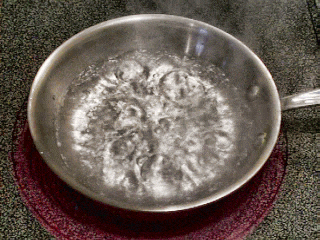# Heat of Vaporization

$$\newcommand{\vecs}{\overset { \rightharpoonup} {\mathbf{#1}} }$$ $$\newcommand{\vecd}{\overset{-\!-\!\rightharpoonup}{\vphantom{a}\smash {#1}}}$$$$\newcommand{\id}{\mathrm{id}}$$ $$\newcommand{\Span}{\mathrm{span}}$$ $$\newcommand{\kernel}{\mathrm{null}\,}$$ $$\newcommand{\range}{\mathrm{range}\,}$$ $$\newcommand{\RealPart}{\mathrm{Re}}$$ $$\newcommand{\ImaginaryPart}{\mathrm{Im}}$$ $$\newcommand{\Argument}{\mathrm{Arg}}$$ $$\newcommand{\norm}{\| #1 \|}$$ $$\newcommand{\inner}{\langle #1, #2 \rangle}$$ $$\newcommand{\Span}{\mathrm{span}}$$ $$\newcommand{\id}{\mathrm{id}}$$ $$\newcommand{\Span}{\mathrm{span}}$$ $$\newcommand{\kernel}{\mathrm{null}\,}$$ $$\newcommand{\range}{\mathrm{range}\,}$$ $$\newcommand{\RealPart}{\mathrm{Re}}$$ $$\newcommand{\ImaginaryPart}{\mathrm{Im}}$$ $$\newcommand{\Argument}{\mathrm{Arg}}$$ $$\newcommand{\norm}{\| #1 \|}$$ $$\newcommand{\inner}{\langle #1, #2 \rangle}$$ $$\newcommand{\Span}{\mathrm{span}}$$$$\newcommand{\AA}{\unicode[.8,0]{x212B}}$$

Because the molecules of a liquid are in constant motion and possess a wide range of kinetic energies, at any moment some fraction of them has enough energy to escape from the surface of the liquid to enter the gas or vapor phase. This process, called vaporization or evaporation, generates a vapor pressure above the liquid. The Heat of Vaporization (also called the Enthalpy of Vaporization) is the heat required to induce this phase change.Figure $$\PageIndex{1}$$: Heat imparts energy into the system to overcome the intermolecular interactions that hold the liquid together to generate vapor.

Since vaporization requires heat to be added to the system and hence is an endothermic process, therefore $$\Delta H_{vap} > 0$$ as defined:

$\Delta H_{vap} = H_{vapor} - H_{liquid}$

where

• $$\Delta H_{vap}$$ is the change in enthalpy of vaporization
• $$H_{vapor}$$ is the enthalpy of the gas state of a compound or element
• $$H_{liquid}$$ is the enthalpy of the liquid state of a compound or element

Heat is absorbed when a liquid boils because molecules which are held together by intermolecular attractive interactions and are jostled free of each other as the gas is formed. Such a separation requires energy (in the form of heat). In general the energy needed differs from one liquid to another depending on the magnitude of the intermolecular forces. We can thus expect liquids with strong intermolecular forces to have larger enthalpies of vaporization. The list of enthalpies of vaporization given in the Table T5 bears this out.

##### Example $$\PageIndex{1}$$

If a liquid uses 50 Joules of heat to vaporize one mole of liquid, then what would be the enthalpy of vaporization?

###### Solution

The heat in the process is equal to the change of enthalpy, which involves vaporization in this case

$q_{tot} = \Delta_{vap}$

so

$q_{tot} = 50 \; J= \Delta_{vap}$

So the enthalpy of vaporization for one mole of substance is 50 J.

##### Kinetic energy does not change

The kinetic energy of the molecules in the gas and the silquid are the same since the vaporization process occues at constant temperature. However, the add thermal energy is used to break the potential energies of the intermolecular forces in the liquid, to generate molecules in the gas that are free of potential energy (for an ideal gass). Thus, while $$H_{vapor} > H_{liquid}$$, the kinetic energies of the molecules are equal.

## The Enthalpy of Condensation

Condensation is the opposite of vaporization, and therefore $$\Delta H_{condensation}$$ is also the opposite of $$\Delta H_{vap}$$. Because $$\Delta H_{vap}$$ is an endothermic process, where heat is lost in a reaction and must be added into the system from the surroundings, $$\Delta H_{condensation}$$ is an exothermic process, where heat is absorbed in a reaction and must be given off from the system into the surroundings.

\begin{align} ΔH_{condensation} &= H_{liquid} - H_{vapor} \\[4pt] &= -ΔH_{vap} \end{align}

Because $$ΔH_{condensation}$$, also written as $$ΔH_{cond}$$, is an exothermic process, its value is always negative. Moreover, $$ΔH_{cond}$$ is equal in magnitude to $$ΔH_{vap}$$, so the only difference between the two values for one given compound or element is the positive or negative sign.

##### Example $$\PageIndex{2}$$

2.055 liters of steam at 100°C was collected and stored in a cooler container. What was the amount of heat involved in this reaction? The $$ΔH_{vap}$$ of water = 44.0 kJ/mol.

###### Solution

1. First, convert 100°C to Kelvin.

°C + 273.15 = K
100.0 + 273.15 = 373.15 K

2. Find the amount involved (in moles).

\begin{align*} n_{water} &= \dfrac{PV}{RT} \\[4pt] &= \dfrac{(1.0\; atm)(2.055\; L)}{(0.08206\; L\; atm\; mol^{-1} K^{-1})(373.15\; K)} \\[4pt] &= 0.0671\; mol \end{align*}

3. Find $$ΔH_{cond}$$

$ΔH_{cond} = -ΔH_{vap} \nonumber$

so

$ΔH_{cond} = -44.0\; kJ/ mol \nonumber$

4. Using the $$ΔH_{cond}$$ of water and the amount in moles, calculate the amount of heat involved in the reaction. To find kJ, multiply the $$ΔH_{cond}$$ by the amount in moles involved.

\begin{align*} (ΔH_{cond})(n_{water}) &= (-44.0\; kJ/mol)(0.0671\; mol) \\[4pt] &= -2.95\; kJ \end{align*}

## Definitions of Terms

Vaporization (or Evaporation) the transition of molecules from a liquid to a gaseous state; the molecules on a surface are usually the first to undergo a phase change.

• Enthalpy: the amount of heat consumed or released in a system at constant pressure
• Kinetic Energy: the energy of a moving object measured in Joules
• Endothermic: when heat is added to a system from the surroundings, due to a decrease in heat in a reaction
• Exothermic: when heat from a system is given off into its surroundings, due to the increase in heat in a reaction
• Heat of Vaporization: the amount of heat required to evaporate a liquid
• System and its Surroundings: the system is the area in which a reaction takes place and the surroundings is the area that interacts with the system
• Condensation: the transition of molecules from a gaseous or vapor state to a liquid
• State Function (or Function of State): any property, such as temperature, pressure, enthalpy or mass, that has a unique value or number for the specific state of a system

## References

1. Petrucci, Ralph H., William S. Harwood, F. G. Herring, and Jeffry D. Madura. General Chemistry: Principles & Modern Applications. 9th ed. Upper Saddle River, NJ: Pearson Prentice Hall, 2007. 474.